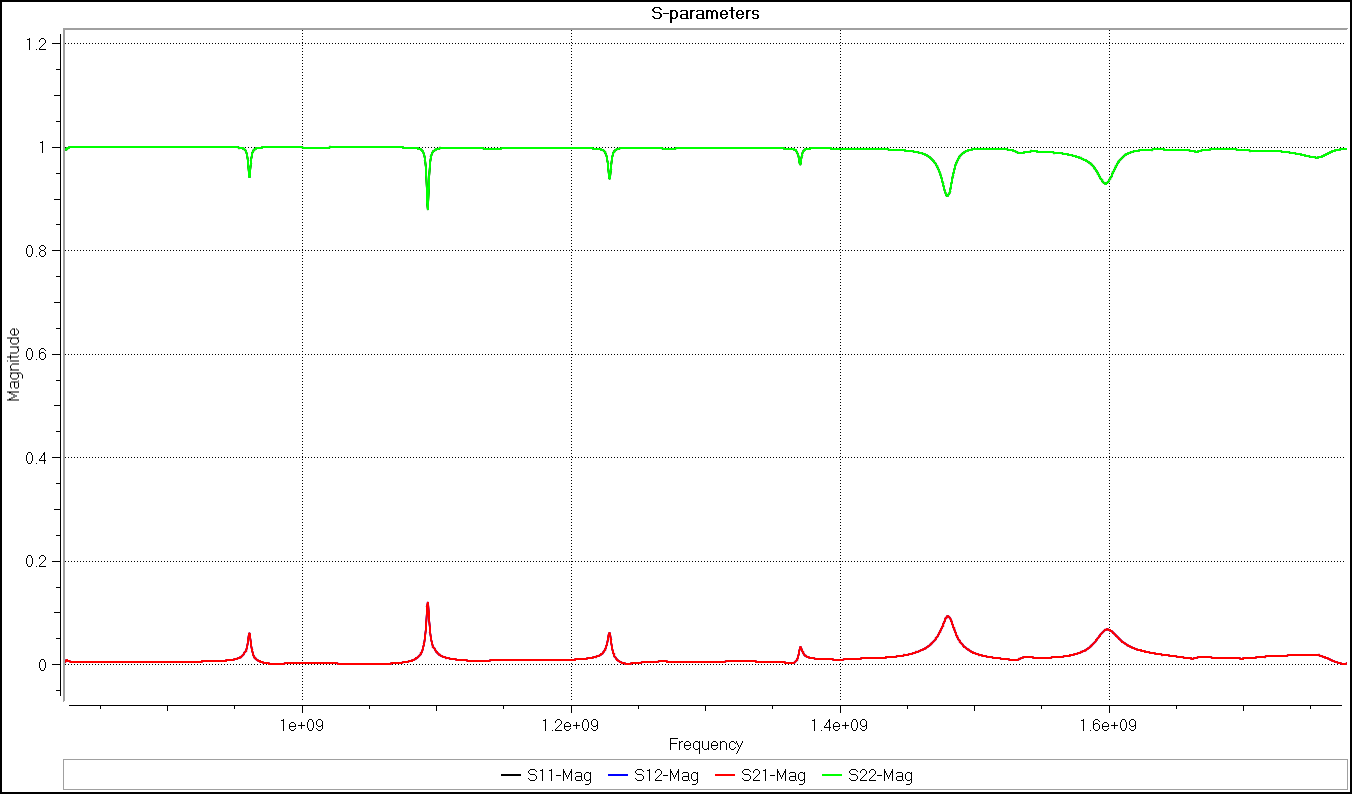# S-Parameters

Scattering parameters (S-parameters) represent the way voltage waves propagate through an electrical network. High frequency linear networks can be fully characterised by these parameters which are measured at the ports of the network. S-parameters are a particularly useful measurement tool as there is no need to identify the components in the network to characterise it.

## Two-Port Network Theory

Two-port networks are often used to describe how S-parameters are calculated, although S-parameters can be used to characterise electrical networks with any number of ports. The theory remains the same for different numbers of ports, only the number of parameters change. There are always N2 S-parameters for an N-port network, each one representing a potential input-output path. S-parameters are complex numbers. In a two port network there are four S-parameters.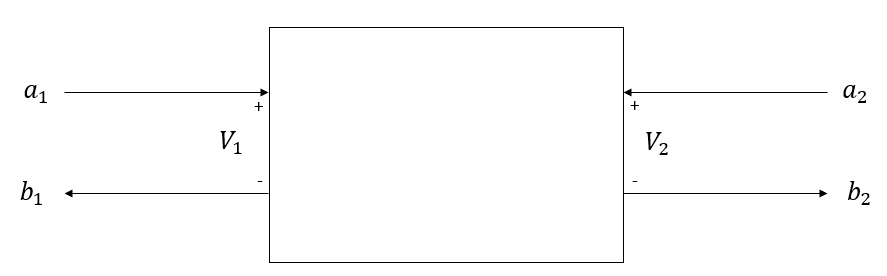In linear networks, when a voltage wave is incident on a port, the energy splits up:

• some energy goes into the port and exists
• some energy goes into the port and scatters to the other ports
• some energy goes into the port and is reflected back out

S-parameters represent the voltage ratios in the network and are are usually displayed in a matrix format. The first and second subscript numbers in an S-parameter for a two-port network is the output port number and the input port number respectively. The relationship between the incident voltage waves and the reflected waves is given by equation: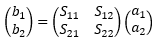(1)

Where the variables are described as:

• a1 - voltage wave incident on port 1
• a2 -voltage wave incident on port 2
• b1 - voltage wave reflected from port 1
• b2 - voltage wave reflected from port 2
• S11 - input reflection coefficient
• S12 - reverse voltage gain
• S21 - forward voltage gain
• S22 - output reflection coefficient

## Measuring S-parameters

S-parameters are typically measured using a voltage source and a matched load Z0 (load with the same value as system impedance). S-parameters are measured by successively opening and shorting different input/output ports of the network. The matrix in the equation above can be expanded to give the two equations: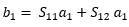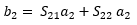(2)

These equations can then be rearranged for each S-parameter and using the maximum power transfer theorem (inserting a matched load at a port sets that ports voltage to zero), the equations for the parameters can be calculated using the following equations: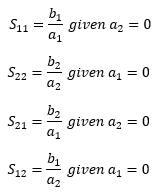(3)

So for example, to calculate the input reflection coefficient of port 1 (S11), the user would apply a voltage across the port 1, place a matched load at (or ground) a2, then measure the voltage across band a1. Dividing b1 by a1 gives the input reflection coefficient at port 1.

## Example

The following example demonstrates how to extract the S-parameters from a two-port SAW in OnScale.

This SAW model has two ports each made up of two finger pairs.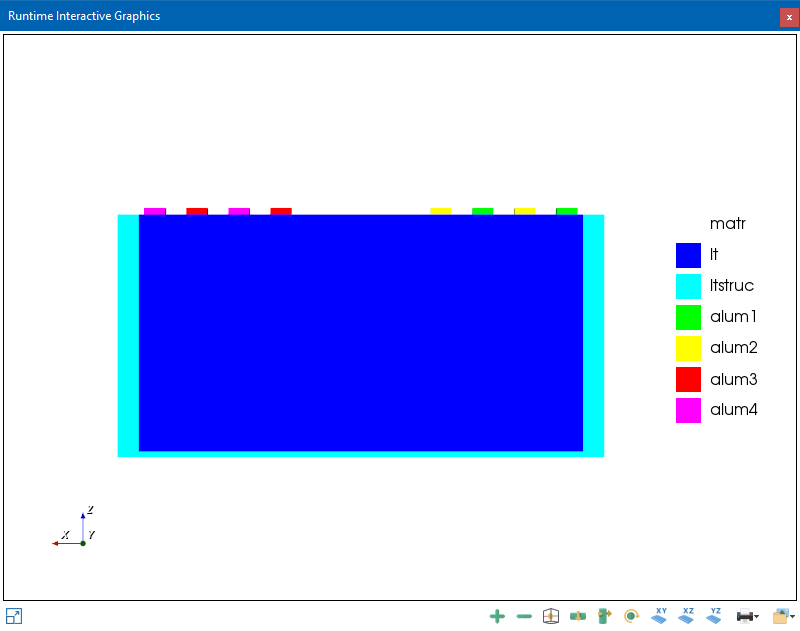To calculate the model's S-parameters, two simulations must be run to acquire all of the information to solve the set of equations (3):

• one that drives port 1 and grounds port 2 (saw_p1.flxinp)
• one that drives port 2 and grounds port 1 (saw_p2.flxinp)
Both simulations output the voltage and charge information (from ports 1 and 2) to history files. Once the simulations are run you can load these files into the Post Processor to easily extract S-parameters using the following steps:
1. Open the post processing tool
2. Load in both time histories (saw_p1.flxhst and saw_p2.flxhst) by double clicking them in File Explorer
3. Select pize idt1_act:Voltage in the Results Manager
4. Select the S-parameters button in the Home tab of the ribbon
5. Assign time histories to the appropriate records as follows: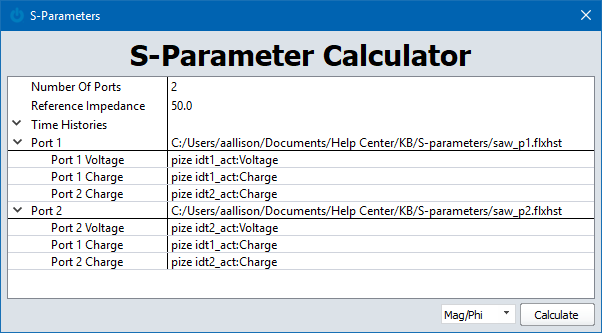6. Select Calculate
7. The results will appear in the results manager under the Frequency History section
8. S-parameters can be plot by double clicking on the frequency history
9. You can zoom in by clicking and dragging or setting the xAxis/yAxis Minimum/Maximums in Plot Controls